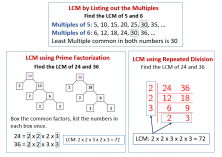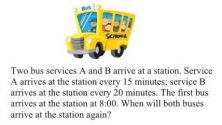Smartick is a fun way to learn math!Dec16

# Interleaving vs Blocked Practice to Learn Math

”What is the difference between interleaving and blocked practice?” We will be writing various entries explaining the difference between these two types of practice and how we use them in Smartick.

### Blocked Practice

In math textbooks and classrooms, it is common for students to study a topic and then, immediately after, apply what they have learned to solve several problems related to that content. For example, after learning about the least common multiple of two numbers several problems using this process will be presented. Problems similar to ”Peter and Mary were both at the park today. Peter goes every 20 days and Mary goes every 24. How many days will pass until they are both at the park again?”This scheme is repeated throughout a child’s time at school. From the start of primary school, students learn to add and then solve addition problems. Then, they learn to subtract and solve subtraction problems. When they begin to mix addition and subtraction problems students know that addition is used to increase and subtraction to decrease. Depending on the situation being described in the problem, students choose between the two. Once they get to multiplication, which also increases the result, some students ask, ”Is this addition or multiplication?” This question shows that students acquire mathematical knowledge about arithmetic operations but find it difficult to determine which operations to use with different problems.

This way of presenting exercises and math problems is known as blocked practice. Organizing exercises in this manner often generates expectations for students which limits their learning in mathematics. When a student faces a problem they often think they need to apply the knowledge that they have just studied. ”If we are doing subtraction today, then they will do subtraction problems; if we learn about division, we will do division problems, etc.”

When using blocked practice students learn the mathematical content but in the long term will most likely experience difficulties solving problems. Why does this happen? In math, exercises and problems tend to be distinguished. Exercises are tasks where you know what process to use to find the solution. However, problems are more complex and require higher mathematical thinking by selecting an adequate strategy to find a solution. When a problem becomes mastered in such a way that it doesn’t require reflection and is solved in a more automatic way, it stops being a problem and becomes an exercise.

In the long term, blocked practice does not provide a sufficient amount of learning opportunities for students to know when to apply each math topic. It is not a very successful method to use when teaching how to choose strategies to solve a problem. When the time comes for the final exam and the problems appear all mixed together, teachers will notice which students solve exercises well but have a hard time with problems.

### Interleaving (mixed practice)

Interleaving consists of mixing math problems together so that two consecutive problems cannot be solved using the same strategy or in the same way. This type of practice ”forces” the student to think of which strategy or procedure to use to solve each problem. To think about the problem itself and not trick themselves and use what they have just studied in class. Interleaving is the kind of practice most similar to an exam situation, where problems are mixed together. This type of practice shows when a student not only knows the procedures but also when to use them.

At Smartick, we dedicate the final minutes of each daily session to working on math problems that use the interleaving method. Additionally, the problems given are not related to the content presented at the beginning of the session. This is to ensure that our students do not develop inaccurate strategies such as, ‘this problem is addition because we worked on addition during the session.

This is not the only way that we apply interleaving in Smartick activities. In the coming blog posts, we will explain other ways to apply these principles of mixed practice to achieve meaningful learning.

Fun is our brain’s favorite way of learning
Diane Ackerman
Smartick is a fun way to learn math
• 15 fun minutes a day